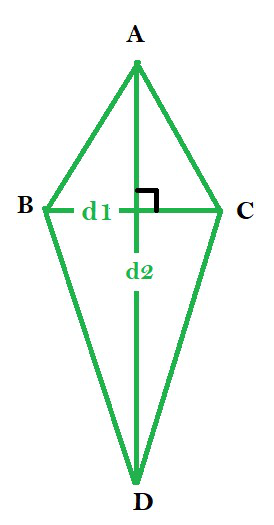# Area of a Kite Formula

• Last Updated : 01 Jan, 2022

Quadrilaterals can be defined as, types of polygons that have four sides, four vertices, and four angles along with a pair of diagonals. The Sum of interior angles of quadrilaterals is 360°. There are various kinds of quadrilaterals. As the name itself suggest the word is a combination of two Latin words ‘Quadri‘ means a variant of four, and ‘latus‘ means side. There are some special types of parallelogram like rectangle, square, rhombus, kite, etc.

A kite is defined as a quadrilateral whose four sides are divided into two pairs, where each pair has two alternate sides of equal length. The elements of a kite are four angles, four sides, and two diagonals.Properties

• The sides are divided into two pairs whose each pair has adjacent sides of equal length.

AB = AC and BD = CD

• The angles of the two vertices connecting the unequal sides are equal.

∠B = ∠C

• The diagonals of a kite intersect at right angles.
• The diagonal joining the two equal angles gets bisected by the other diagonal of the kite.
• The perimeter of the kite is twice the sum of unequal sides.

Perimeter = 2 × (AB + BD) = 2 × (AC + CD)

### Area of a kite

The area of a kite is given as half of the product of the length of the two diagonals of the kite.

Area of a kite = 1/2 × d1 × d2

where,

d1 = shorter diagonal of the kite

d2 = longer diagonal of the kite

Proof:

Consider the image shown above. There are two triangles formed namely ACD and ABD.

Since diagonal d1 is bisected by diagonal d2, the altitudes of both the triangles are equal to (d1)/2.

The common base of triangles ACD and ABD is AD.

Thus, the length of the base = Length of AD = d2

The area of a triangle is given by:

Area of triangle = 1/2 × base × altitude

For the kite ABCD, we can write

Area of kite ABCD = Area of triangle ACD + Area of triangle ABD

Area of kite ABCD = 1/2 × d2 × (d1)/2 + 1/2 × d2 × (d1)/2 = 1/2 × d2 × d1

Thus, we can write

Area of kite ABCD = 1/2 × d1 × d2

### Sample Problems

Question 1. Find the area of a kite whose length of diagonals is 5 cm and 6 cm.

Solution:

We know the area of a kite is equal to half of the product of both the diagonals.

Given, d1 = 5cm and d2 = 6cm

Thus, we can write,

Area of the kite = 1/2 × 5 × 6 = 5 × 3 = 15 cm2

Question 2. Rahul wants to buy a kite-shaped plot of area 70 square yards. Given the length of a diagonal of the plot is 20 yards, find the length of the other diagonal of the plot.

Solution:

We know the area of a kite is equal to half of the product of both the diagonals.

Given the length of a diagonal is 20 yards, and the area is 70 square yards.

Let the length of the other diagonal be ‘d‘ yards. Then we can write

70 = 1/2 × 20 × d

70 = 10 × d

d = 70/10 = 7 yards

Thus, the length of the other diagonal is 7 yards.

Question 3. Find the diagonals of a kite, given the sum of the diagonals, is 9 units and area is 10 square units.

Solution:

Given, the area is 10 square units and the sum of diagonals is 9 units.

Let the diagonals be d1 and d2. Then we can write

d1 + d2 = 9

or, d2 = 9 – d1   —- (i)

1/2 × d1 × d2 = 10   —- (ii)

Replacing the value of d2 in eq.(ii), we get

1/2 × d1 × (9 – d1) = 10

d1 × (9 – d1) = 20

d12 – 9d1 + 20 = 0

d12 – 5d1 – 4d1 + 20 = 0

d1(d1 – 5) – 4(d1 – 5) = 0

(d1 – 5) × (d1 – 4) = 0

d1 = ‘5 units’ or ‘4 units’

If the value of d1 is 5 units, then d2 = 9 – d1 = 9 – 5 = 4 units

Else if the value of d1 is 4 units, then d2 = 9 – d1 = 9 – 4 = 5 units

Hence the length of the diagonals are 4 units and 5 units.

Question 4. Find the relation between the area and smaller diagonal of a kite, given the larger diagonal, is twice the smaller diagonal.

Solution:

Lets the smaller and larger diagonal be d and 2d respectively.

Let the area of the kite be A.

Then we can write,

A = 1/2 × d × (2d)

A = d2

Thus, the area of the kite is square of the smaller diagonal.

My Personal Notes arrow_drop_up